# Area of a Triangle - Examples

Example 1: Find the area of an acute triangle with a base of 15 inches and a height of 4 inches.

Solution: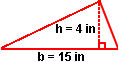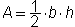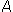=· (15 in) · (4 in)=· (60 in2)= 30 in2

Example 2: Find the area of a right triangle with a base of 6 centimeters and a height of 9 centimeters.Solution:=· (6 cm) · (9 cm)=· (54 cm2)= 27 cm2

Example 3: Find the area of an obtuse triangle with a base of 5 inches and a height of 8 inches.

Solution: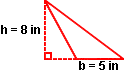=· (5 in) · (8 in)=· (40 in2)= 20 in2

Example 4: The area of a triangle shaped mat is 18 square feet and the base is 3 feet. Find the height. (Note: The triangle in the illustration to the right is NOT drawn to scale.)

Solution:

In this example, we are given the area of a triangle and one dimension, and we are asked to work backwards to find the other dimension.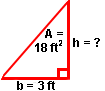18 ft2 =\B7 (3 ft) · h

Multiplying both sides of the equation by 2, we get:

36 ft2 = (3 ft) · h

Dividing both sides of the equation by 3 ft, we get:

12 ft = h

Commuting this equation, we get:

h = 12 ft

Summary: Given the base and the height of a triangle, we can find the area. Given the area and either the base or the height of a triangle, we can find the other dimension. The formula for area of a triangle is:or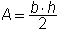where b is the base and h is the height.